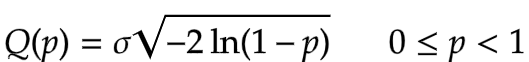# Rayleigh Distribution Calculator

Created by Anna Szczepanek, PhD
Reviewed by Dominik Czernia, PhD and Jack Bowater
Last updated: Jan 16, 2023

Welcome to the Rayleigh distribution calculator! With its help, you can quickly compute a bunch of things related to the Rayleigh distribution. Do you need to find some probabilities, determine some pdf / cdf / quantile values, or just compute the mean and variance of the Rayleigh distribution? Our Rayleigh distribution calculator can do all of this, and even more: it can also draw samples from the Rayleigh distribution!

What is the Rayleigh distribution? To learn more about the Rayleigh distribution function, scroll down! Apart from the Rayleigh distribution definition, we provide formulas for the Rayleigh distribution mean, variance, and other measures!

## What is the Rayleigh distribution?

The Rayleigh distribution is a continuous probability distribution. It owes its name to the English Lord Rayleigh (John William Strutt, 3rd Baron Rayleigh), who encountered this distribution when working on problems in acoustics.

The Rayleigh distribution is a special case of the Weibull distribution, therefore, whatever you know about
the Weibull distribution applies to the Rayleigh distribution
.

Recall that the well-known exponential distribution is also a special case of the Weibull distribution. It turns out that the Rayleigh and exponential distributions are tightly linked: the square root of an exponential random variable is a Rayleigh random variable.

A natural situation where you can encounter the Rayleigh distribution function is when you take two independent random variables, X and Y, that follow the normal distribution with a mean of zero and equal standard deviations. Then the random variable √(X² + Y²) follows the Rayleigh distribution. Please check out our normal distribution calculator.

As we now know what the Rayleigh distribution is, it is time to explain how you can use our Rayleigh calculator. In the following sections, you will find the definition of the Rayleigh distribution and a bunch of formulas related to it, including that of the Rayleigh cumulative distribution function (cdf of Rayleigh distribution) and the Rayleigh probability density function (pdf of Rayleigh distribution).

## How to use this Rayleigh calculator?

To use the Rayleigh calculator, follow the instruction below:

1. Choose the calculator mode. Our Rayleigh distribution calculator has several modes. Thanks to them, you can very quickly generate samples from the Rayleigh distribution or determine:

• Probabilities;
• Values of the cumulative distribution function;
• Values of the probability density function;
• Values of the quantile function; and
• Common measures of the Rayleigh distribution.

In particular, if you want to find the mean and variance of the Rayleigh distribution, you should use the common measures mode.

2. For each Rayleigh distribution function, enter the value of the parameter you want to use in the calculations.

3. Depending on the mode you've chosen, provide any additional input. For instance, for the cdf mode you need to enter the argument x at which you want to evaluate the Rayleigh cdf.

4. That's all! The Rayleigh distribution calculator displays the result immediately!

## Rayleigh distribution function and definition

The probability density function (pdf) of the Rayleigh distribution reads:where σ > 0 is a parameter. Let's see what the pdf of the Rayleigh distribution looks like for different Rayleigh distribution examples with various values of σ:

The cumulative distribution function (cdf) of the Rayleigh distribution is the following:Here you can see what the cdf of the Rayleigh distribution looks like for different Rayleigh distribution examples with various σ:

We can easily derive the quantile function (i.e., the inverse of the cdf) of the Rayleigh distribution. It's given by the following formula:## Variance and mean of the Rayleigh distribution (and other measures)

This section provides the formulae for the common measures of the Rayleigh distribution: its mean, median, mode, variance, and skewness.

• Mean of the Rayleigh distribution:

σ * √(π / 2)

• Median of the Rayleigh distribution:

σ * √(2 ⋅ ln(2))

• Mode of the Rayleigh distribution:

σ

• Variance of the Rayleigh distribution:

σ² * (4 - π)/2

Obviously, the standard deviation of the Rayleigh distribution is just the square root of the Rayleigh variance.

• Skewness of the Rayleigh distribution:

2 * (π - 3) * √(π / (4 - π)³)

Please check out our median calculator, mode calculator, variance calculator and skewness calculator to understand more on this topic.

## Applications of the Rayleigh distribution - Rayleigh distribution examples

The Rayleigh distribution appears repeatedly in mathematical modeling. For instance, you can encounter it in:

• Electrical engineering - to model the lifetime of resistors, capacitors and transformers;
• Physics - to model sound or light radiation;
• Weather forecasting - to model wind speed; AND
• Hydrology - to model wave heights
Anna Szczepanek, PhD
Mode
Probability calculator
Probability type
P(X ≤ x)
Scale parameter, σ
Argument, x
P(X ≤ x)
People also viewed…

Addiction calculator tells you how much shorter your life would be if you were addicted to alcohol, cigarettes, cocaine, methamphetamine, methadone, or heroin.

### Car heat

The hot car calculator shows how fast a car's interior heats up during a summer day.

### Midrange

The midrange calculator will quickly calculate the midrange of a given set of data.

### Sampling distribution of the sample proportion

Use our sampling distribution of the sample proportion calculator to find the probability that your sample proportion falls within a range.